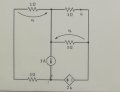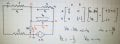# Finding the voltage V2 and V6 using the node method.

Joined Dec 17, 2022
7
Good evening, I am trying to complete this exercise where I am asked to find the value of voltage V2 and V6.

I have used the node method but I always get stuck at this final point, I can't go on.

Could someone kindly help me?

I include photos.This, however, is what I did:I know from some colleagues that the results must be: V2=-1/3
and V6=-4, what I cannot understand are the intermediate steps, how do we arrive at this solution?

#### RBR1317

Joined Nov 13, 2010
712
I tried to simplify the original diagram, but might be confused by the drawing/labeling conventions that were used.

#### WBahn

Joined Mar 31, 2012
29,510
I'm confused by your work. You say that this is the work you did. While I don't quite see how you came up with the matrices that you did, but you seem to have come up with what you claim others told you is the correct result. How can you not know how the work you did resulted in the answers you got?

Because you have a voltage source and are trying to do nodal analysis, it is often worthwhile choosing one terminal of the voltage source as your 0 V reference.

If you choose the right-hand node (what you called B) as the reference, that makes your two node equations easy to write down. One of them is trivial.

That will allow you to directly solve for the voltage on the top-middle node (the one you chose as your reference), followed immediately the voltage on Node A. The top-middle node is V6 and you can use the voltage on the two middle nodes to find the current in the 1 Ω resistor, which then gives you V2.

#### The Electrician

Joined Oct 9, 2007
2,953
what I cannot understand are the intermediate steps, how do we arrive at this solution?
Look carefully at your dependent voltage source. The current through it is 2*i4, and the voltage across it due to that current is -2*i4. Ask yourself what electronic component has the property that the voltage across it is equal to the negative of the current through it? It is equivalent to a -1Ω resistor. Replace the dependent source with a -1Ω resistor and the circuit becomes trivial to solve using nodal analysis or just plain old Ohm's law.

#### MrAl

Joined Jun 17, 2014
10,906
Good evening, I am trying to complete this exercise where I am asked to find the value of voltage V2 and V6.

I have used the node method but I always get stuck at this final point, I can't go on.

Could someone kindly help me?

I include photos.

View attachment 297602

This, however, is what I did:

View attachment 297603

I know from some colleagues that the results must be: V2=-1/3
and V6=-4, what I cannot understand are the intermediate steps, how do we arrive at this solution?

Hello there,

Nodal analysis is a general way to solve circuit problems. It is based mostly on the sum of currents entering a node is equal to the sum leaving the node, or the sum of currents entering or leaving a node is equal to zero. You probably have to get that part right first.
Voltage sources are treated as special kinds of shorts called super nodes. I don't prefer that name however because it is too nondescript, so it doesn't really say anything about what it is. A better name is aniselectric short or something along those lines. it simply means that the voltage source is one of two possible things depending on what you are solving for or measuring:
1. It is a short for current.
2. It is a voltage source for voltage.
What this means is that the sum of CURRENTS into B is both the sum from both resistors connected to B added to the sum of CURRENTS at A, because B is shorted to A.
The dual nature of this kind of short is evident when we realize that the VOLTAGE across A,B is still 2*i4 it is not zero.

BTW it's always a good idea to label the resistors R1, R2, R3, etc. so others can refer to the specific components.

That being said, the first thing you would do is try to simplify the network by combining any elements that are not hard to combine, such as series resistors and parallel resistors. That makes the circuit easier to comprehend right away.